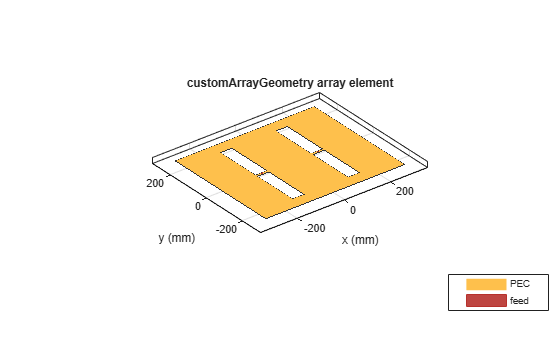# customArrayGeometry

Create array represented by 2-D custom geometry

## Description

The `customArrayGeometry` object is an array represented by a 2-D custom geometry on the xy-plane. You can use the `customArrayGeometry` to import a 2-D custom geometry, define feeds to create an array element, and analyze the custom array.

## Creation

### Syntax

``array = customArrayGeometry``
``array = customArrayGeometry(Name=Value)``

### Description

``` `array = customArrayGeometry` creates a custom array represented by 2-D geometry on the X-Y plane, based on the specified boundary.```

example

``` `array = customArrayGeometry(Name=Value)` creates a 2-D array geometry, with additional Properties specified by one or more name-value arguments. `Name` is the property name and `Value` is the corresponding value. You can specify several name-value arguments in any order as `Name1=Value1`, `...`, `NameN=ValueN`. Properties not specified retain their default values.```

### Output Arguments

expand all

Custom array geometry, returned as an `customArrayGeometry` object.

## Properties

expand all

Boundary information in Cartesian coordinates, specified as a cell array in meters.

Data Types: `double`

Boolean operation performed on the boundary list, specified as a character vector. operation set is; [`+`, `-`, `*`].

Example: `'P1-P2'`

Data Types: `char`

Array element feed location in Cartesian coordinates, specified as a three-element vector. The three elements represent the X, Y, and Z coordinates respectively.

Example: `[0 0.2 0]`

Data Types: `double`

Width of feed for array elements, specified as a scalar in meters.

Example: `'FeedWidth',0.05`

Data Types: `double`

Excitation amplitude for array elements, specified as a positive scalar or vector. Set property value to `0` to model dead elements.

Example: `3`

Data Types: `double`

Phase shift for array elements, specified as a real scalar or a real vector in degrees.

Example: `[3 3 0 0]` specifies the phase shift for custom array containing four elements.

Data Types: `double`

Tilt angle of the array in degrees, specified as a scalar or vector. For more information, see Rotate Antennas and Arrays.

Example: `90`

Example: `Tilt=[90 90]`,`TiltAxis=[0 1 0;0 1 1]` tilts the array at 90 degrees about the two axes, defined by the vectors.

Data Types: `double`

Tilt axis of the array, specified as one of these values:

• Three-element vectors of Cartesian coordinates in meters. In this case, each vector starts at the origin and lies along the specified points on the x-, y-, and z-axes.

• Two points in space, specified as a 2-by-3 matrix corresponding to two three-element vectors of Cartesian coordinates. In this case, the array rotates around the line joining the two points.

• `"x"`, `"y"`, or `"z"` to describe a rotation about the x-, y-, or z-axis, respectively.

Example: `[0 1 0]`

Example: `[0 0 0;0 1 0]`

Example: `"Z"`

Data Types: `double` | `string`

## Object Functions

 `show` Display antenna, array structures, shapes, or platform `info` Display information about antenna or array `axialRatio` Axial ratio of antenna `beamwidth` Beamwidth of antenna `charge` Charge distribution on antenna or array surface `current` Current distribution on antenna or array surface `efficiency` Radiation efficiency of antenna `EHfields` Electric and magnetic fields of antennas or embedded electric and magnetic fields of antenna element in arrays `impedance` Input impedance of antenna or scan impedance of array `mesh` Mesh properties of metal, dielectric antenna, or array structure `meshconfig` Change meshing mode of antenna, array, custom antenna, custom array, or custom geometry `pattern` Plot radiation pattern and phase of antenna or array or embedded pattern of antenna element in array `patternAzimuth` Azimuth plane radiation pattern of antenna or array `patternElevation` Elevation plane radiation pattern of antenna or array `returnLoss` Return loss of antenna or scan return loss of array `sparameters` Calculate S-parameters for antennas and antenna arrays `show` Display antenna, array structures, shapes, or platform `vswr` Voltage standing wave ratio (VSWR) of antenna or array element

## Examples

collapse all

Create and visualize a custom array using `customArrayGeometry`. Plot the impedance and current distribution of the array.

Create a ground plane with a length of 0.6 m and a width of 0.5 m.

```Lp = 0.6; Wp = 0.5; p1 = antenna.Rectangle(Length=Lp, Width=Wp);```

Create slots of length 0.05 m and width 0.4 m on the ground plane.

Add strips of length 0.05 m and width 0.01 m in between the slots for housing the feed point.

```Ls = 0.05; Ws = 0.4; offset = 0.12; p2 = antenna.Rectangle(Length=Ls, Width=Ws, Center=[-offset 0]); p3 = antenna.Rectangle(Length=Ls, Width=Ws, Center=[offset 0]); Wf = 0.01; p4 = antenna.Rectangle(Length=Ls, Width=Wf, Center=[-offset 0]); p5 = antenna.Rectangle(Length=Ls, Width=Wf, Center=[offset 0]);```

Create custom array using the slotted ground plane. Create a feed in between the slots on the ground plane.

```b1 = getShapeVertices(p1); b2 = getShapeVertices(p2); b3 = getShapeVertices(p3); b4 = getShapeVertices(p4); b5 = getShapeVertices(p5); carray = customArrayGeometry; carray.Boundary = {b1,b2,b3,b4,b5}'; carray.Operation = 'P1-P2-P3+P4+P5'; carray.NumFeeds = 2; carray.FeedLocation = [-offset 0 0; offset 0 0]; carray.FeedWidth = [Wf Wf];```

Visualize the array.

```figure show(carray)```Calculate the impedance of the array using the frequency range of 350 MHz to 450 MHz.

```figure impedance(carray, 350e6:5e6:450e6)```Visualize the current distribution of the array at 410 MHz.

```figure current(carray, 410e6, Scale="log10")```Balanis, C. A. Antenna Theory. Analysis and Design. 3rd Ed. Hoboken, NJ: John Wiley & Sons, 2005.